Q:

# Program to convert hours into minutes and seconds

In this article, we will discuss the programs to convert the hours into minutes and seconds in different programming languages.

But before writing the programs, let's first see a brief description of converting the hours into minutes and seconds.

### How to convert the hours into minutes and seconds?

We know that there are 60 minutes in an hour, and there are 60 seconds in a minute. But here, we only have the value of hours as an input. We have to convert that input into minutes and seconds. So, the formula to convert the hours into minutes and seconds is -

minutes = hours x 60

seconds = hours x 3600

Now, let's see the programs to convert the hours into minutes and seconds.

### Programs to convert the hours into minutes and seconds

Program: Write a program to convert the hours into minutes and seconds in C language.

``````#include <stdio.h>
void conversion(float hours)
{
double minutes, seconds;

minutes = hours * 60;
seconds = hours * 3600;

printf("There are %lf minutes in %f hours", minutes, hours);
printf("\nThere are %lf seconds in %f hours", seconds, hours);
}

int main()
{
float hours;
printf("Enter the value of hours: ");
scanf("%f", &hours);
conversion(hours);
return 0;
}  ``````

Output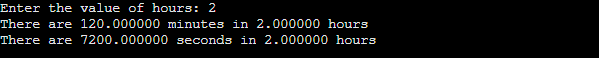Program: Write a program to convert the hours into minutes and seconds in C++ language.

``````#include <iostream>
using namespace std;

void conversion(float hours)
{
double minutes, seconds;

minutes = hours * 60;
seconds = hours * 3600;

cout<<"There are "<< minutes << " minutes in "<< hours <<" hours" <<endl;
cout<<"There are "<< seconds << " seconds in "<< hours <<" hours" <<endl;
}
int main()
{
float hours;
cout<<"Enter the value of hours: ";
cin>>hours;
conversion(hours);

return 0;
}  ``````

Output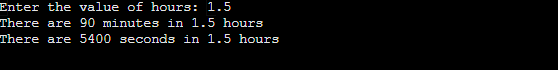Program: Write a program to convert the hours into minutes and seconds in C#.

``````using System;

class JTP
{

static void conversion(float hours)
{
double minutes, seconds;

minutes = hours * 60;
seconds = hours * 3600;

Console.WriteLine("There are " + minutes + " minutes in " + hours + " hours");
Console.WriteLine("There are " + seconds + " seconds in " + hours + " hours");
}
public static void Main ()
{
float hours;
Console.Write("Enter the value of hours: ");
conversion(hours);
}
}  ``````

Output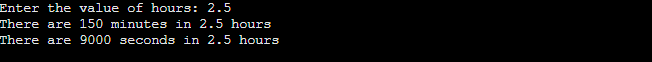Program: Write a program to convert the hours into minutes and seconds in Java.

``````import java.io.*;
import java.util.*;

class Conversion {

static void conversion(float hours)
{
float minutes, seconds;

minutes = hours * 60;
seconds = hours * 3600;

System.out.println("There are " + minutes + " minutes in " + hours + " hours");
System.out.println("There are " + seconds + " seconds in " + hours + " hours");
}
public static void main (String[] args) {
float hours;
System.out.println("Enter the value of hours: ");
Scanner sc = new Scanner(System.in);
hours = sc.nextFloat();
conversion(hours);
}

}  ``````

Output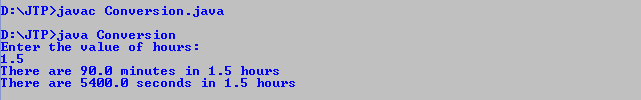Program: Write a program to convert the hours into minutes and seconds in JavaScript.

``````<html>
<body>
<script>
function conversion(hours) {
var minutes, seconds;

minutes = hours * 60;
seconds = hours * 3600;

document.write("Hours = " + hours + "<br>");
document.write("There are " + minutes + " minutes in " + hours + " hours <br>");
document.write("There are " + seconds + " seconds in " + hours + " hours");

}
var hours = prompt("Enter the value of hours: ");
conversion(hours);
</script>

</body>
</html>  ``````

Output

After the execution of the above code, the output will be -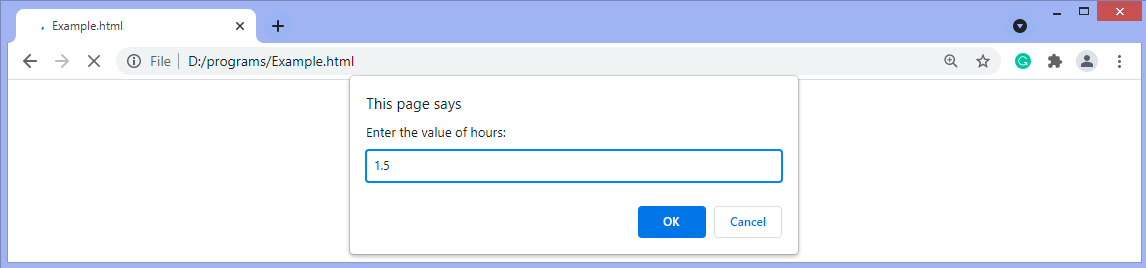After entering the value and clicking OK, the output will be -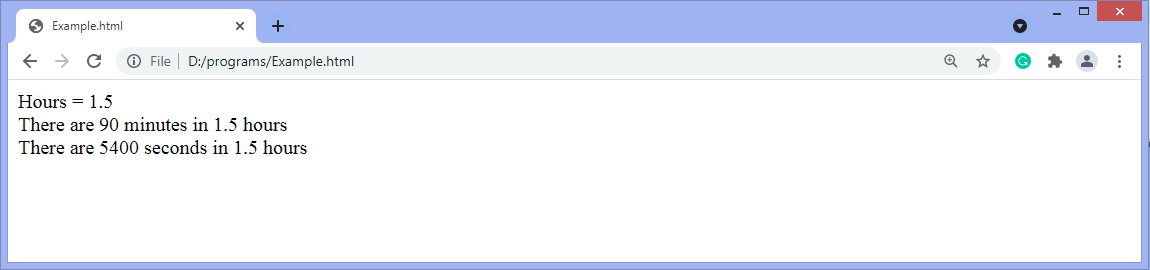Program: Write a program to convert the hours into minutes and seconds in PHP.

``````<?php
function conversion(\$hours)
{
\$minutes = \$hours * 60;
\$seconds = \$hours * 3600;

echo("Hours = " . \$hours . "<br>");
echo("There are " . \$minutes . " minutes in " . \$hours . " hours <br>");
echo("There are " . \$seconds . " seconds in " . \$hours . " hours");
}
\$hours = 3.5;
conversion(\$hours);
?>  ``````

Output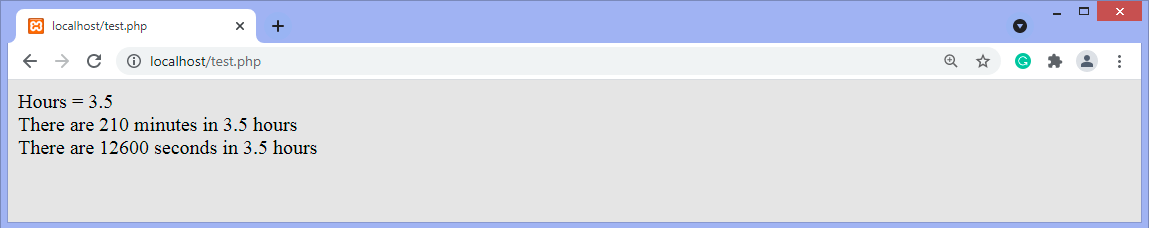Program: Write a program to convert the hours into minutes and seconds in python.

``````def conversion(hours):

minutes = hours * 60;
seconds = hours * 3600;

print("There are " , minutes , " minutes in " , hours , " hours");
print("There are " , seconds , " seconds in " , hours , " hours");

hours = float(input('Enter the value of hours: '))
conversion(hours);  ``````

Output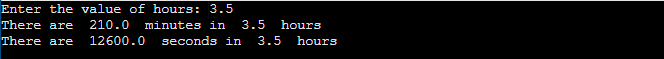So, that's all about the article. Here, we have discussed the programs to convert hours into minutes and seconds in C, C++, C#, Java, JavaScript, PHP and Python. Hope you find the article helpful and informative.